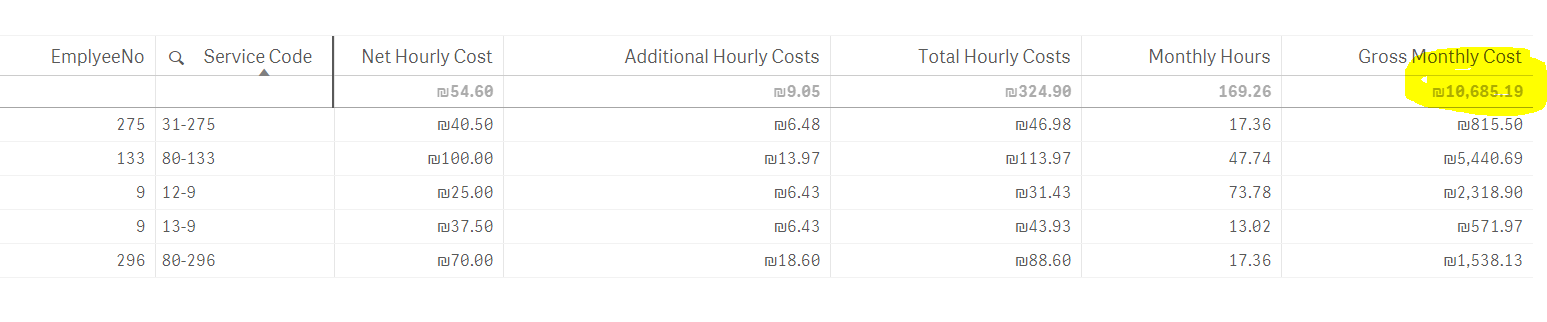# New to Qlik Sense

Discussion board where members can get started with Qlik Sense.

New Contributor III

## Can't get KPI to show table column Total result

I am trying to show the Table sum in yellow below (column Gross Monthly Cost) in a KPI but i can't get it work.

This "gross Monthly Cost" column  calculates Total Hourly Costs * Monthly Hours.

Here are the expressions in each column below:

Net Hourly Cost                  -  Sum(EmployeeRate)

Additional Hourly Costs     - \$(vAluyotNilvotLeshaa)

Monthly Hours                    -   Sum([SessionMonthlyDuration(numeric)])

Gross Monthly Cost           -  (\$(vAluyotNilvotLeshaa)+EmployeeRate)*Sum([SessionMonthlyDuration(numeric)])If I enter the last expression SUM(\$(vAluyotNilvotLeshaa)+EmployeeRate)*Sum([SessionMonthlyDuration(numeric)]) in a KPI  I get "-".

How do I get the KPI to show the summary figure in yellow?

1 Solution

Accepted SolutionsMVP

## Re: Can't get KPI to show table column Total result

Ah sorry, you are trying to get the result in a KPI object.

Seems like your calculation needs some advanced aggregation:

Sum(

Aggr(

(\$(vAluyotNilvotLeshaa)+EmployeeRate)*Sum([SessionMonthlyDuration(numeric)])

, EmployeeNo, [Service Code] )

)

3 RepliesMVP

## Re: Can't get KPI to show table column Total result

Try

=(Column(1)+Column(2)) * Column(4)

to reference the other column results in your new expression

If this doesn't work, then please post the definition of variable vAluyotNilvotLeshaa and if possible, a small sample QVF.MVP

## Re: Can't get KPI to show table column Total result

Ah sorry, you are trying to get the result in a KPI object.

Seems like your calculation needs some advanced aggregation:

Sum(

Aggr(

(\$(vAluyotNilvotLeshaa)+EmployeeRate)*Sum([SessionMonthlyDuration(numeric)])

, EmployeeNo, [Service Code] )

)

New Contributor III

## Re: Can't get KPI to show table column Total result

Thanks Stefan! you are my hero!!I tried playing around with AGGR but didn't get it to work. Your expression did the trick. Thank you!